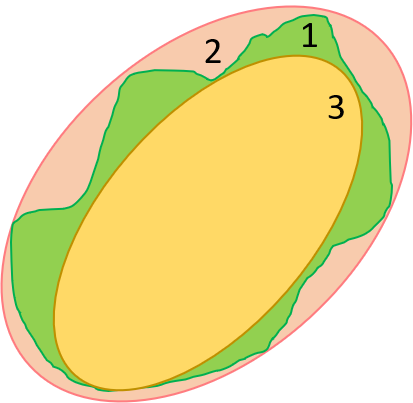# Calculate volume of largest and minimum ellipse around a segmentation

Is there any function in SimpleITK similar to the OpenCV function FitEllipse --> https://docs.opencv.org/3.4.9/de/d62/tutorial_bounding_rotated_ellipses.html ?

What I am trying to do is to calculate the volume of the largest and minimal ellipsoid volume related to a segmented tumor.
Something like this figure, where 1 is my tumor segmentation and 2 and 3 would be the ellipsoid volumes that I need to calculate.

I am not looking for code but just for some direction.I don’t think there is an ellipse fitting class. What you could look into is LabelShapeStatisticsImageFilter and LabelGeometryImageFilter, and possibly their source code.

1 Like

I am not sure how well defined or unique the “minimal” ellipsoid would be.

In the LabelShapeStatisticsImageFilter, the principle axis are computed. There are several derived parameters from that you could look into. The original Insight Journal article may be the best reference.

Beyond those existing attributes you would need to create your own. A method needs to be added to `LabelShapStatisticsImageFilter` to get a list of indices or points for a given label. In the mean time something like `np.where(sitk.GetArrayViewFromImage(lableImage))` could provide similar functionality.

1 Like

Here is the PR adding the new functionality to SimpleITK:

2 Likes

I think I managed to solve this issue.

What is missing is extracting the surface points from a segmentation mask. That could be extracted using the `SimpleITK.LabelContour(img, fullyConnected=False)`.
Also the spacing information is missing, so the ellipsoid should be adjusted by placing it on the correct grid.
This can be achieved `scikit-image.draw.ellipsoid` https://scikit-image.org/docs/dev/api/skimage.draw.html#skimage.draw.ellipsoid.

Is it possible to propose adding this functionality to the official SimpleITK library?

Thanks for sharing your solution. But the problem is somewhat specialized. Moreover, ITK and SimpleITK are written in C++ so you would have to translate your solution into C++ for incorporation.

Thanks for sharing your implementation of computing the optimal ellipsoid.

I believe you are asking to get the points from a contour in SimpleITK.

Here is sample usage:

``````

In : sitk.LabelContour(labels, fullyConnected=False)
Out: <SimpleITK.SimpleITK.Image; proxy of <Swig Object of type 'std::vector< itk::simple::Image >::value_type *' at 0x7f8052bdbc00> >

In : contours = sitk.LabelContour(labels, fullyConnected=False)

In : shape = sitk.LabelShapeStatisticsImageFilter()

In : shape.Execute(contours)

In : shape.GetLabels()
Out: (100, 200)

In : idx = shape.GetIndexes(100)
``````